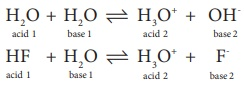# UNIT 8: Ionic Equilibrium - Online Test

Q1. Concentration of the Ag+ ions in a saturated solution of Ag2 C2O4 is 2.24 ×10-4mol L-1 solubility product of Ag2 C2O4 is
Explaination / Solution:

Ag2C2O4 2Ag+ + C2O42-

[ Ag+ ] = 2.24 × 10-4 mol L-1

[ C2 O42- ] = { 2.24×10-4 }/2 = mol L-1

= 1.12×10-4 mol L-1

Ksp = [Ag+]2[C2O42-]

= (2.24×10-4 mol-4 L-1 )2 (1.12×10-4 mol L-1)

= 5.619×10-12mol3 L-3

Q2.

Following solutions were prepared by mixing different volumes of NaOH of HCl different concentrations.

i. 60 mL (M/10) HCl + 40mL (M/10) NaOH

ii. 55 mL (M/10) HCl + 45 mL (M/10) NaOH

iii. 75 mL (M/5) HCl + 25mL (M/5) NaOH

iv. 100 mL (M/10) HCl + 100 mL (M/10) NaOH

pH of which one of them will be equal to 1?
Explaination / Solution:

iii) 75 ml M/5 HCl + 25ml M/5 NaOH

No of moles of HCl = 0.2×75×10-3 = 15 × 10-3

No of moles of NaOH = 0.2 × 25 × 10-3 = 5 × 10-3

No of moles of HCl after mixing = 15 × 10-3 - 5 × 10-3

= 10 × 10-3

concentration of HCl = No of moles of HCl / Vol in litre

= 10 ×103 / 100 ×103 = 0.1M

for (iii) solution, pH of 0.1M HCl = -log10(0.1)

= 1.

Q3. The solubility of BaSO4 in water is 2.42 ×10-3 gL-1 at 298K. The value of its solubility product ( Ksp ) will be. (Given molar mass of BaSO4 =233g mol-1 )
Explaination / Solution:

BaSO4 Ba2+ +SO42-

Ksp =(s) (s)

Ksp =(s)2

= ( 2.42×10-3 g L-1 )2

= ( 2.42×10-3 g L-1 / 233g mol-1 )

= ( 0.01038×10-3 ) 2

= (1.038×10-5 )2

= 1.077×10-10

= 1.08×10-10 mol2 L-2

Q4. pH of a saturated solution of Ca(OH)2 is 9. The Solubility product ( Ksp )of Ca(OH)2
Explaination / Solution:

Ca(OH)2 Ca2+ + 2OH-

Given that pH = 9

pOH = 14-9 = 5

[pOH = -log10 [OH- ]]

[OH- ] = 10- pOH

[OH- ]=10-5 M

Ksp =[Ca2+ ][OH- ]2

= (10-5 /2 )×(10-5 )2

=0.5 ×10-15

Q5. Conjugate base for Bronsted acids H2O and HF are
Explaination / Solution:

H2O + H2O H3O+ + OH-

acid 1 + base 1 acid 2 + base 2HF + H2O H3O+ + F-

acid 1 + base 1 acid 2 + base 2

Conjugate bases are OH- and F- respectively

Q6. Which will make basic buffer?
Explaination / Solution:

Basic buffer is the solution which has weak base and its salt

NH4OH(200ml) + HCl(100ml) NH4Cl(Salt)  + H2O + NH4OH (100ml weak base)

Q7. Which of the following fluro compounds is most likely to behave as a Lewis base?
Explaination / Solution:

BF3 elctron deficient Lewis acid

PF3 electron rich lewis base

CF4 neutral neither lewis acid nor base

SiF4- neutral neither lewis acid nor base

Q8. Which of these is not likely to act as Lewis base?
Explaination / Solution:

BF3 elctron deficient Lewis acid

PF3 electron rich lewis base

CO having lone pair of electron lewis base

F- unshared pair of electron lewis base

Q9. The aqueous solutions of sodium formate, anilinium chloride and potassium cyanide are respectively
Explaination / Solution:

HCOONa Basic in nature. + H OH NaOH strong base  + H-COOH weak acid

C6H5NH3Cl- + H OH H3O+Acidic +C6H5 -NH2 + Cl-

KCN basic + H− OH KOH strong base + HCN weak acid

basic, acidic, basic is correct.

Q10. The percentage of pyridine (C5H5N) that forms pyridinium ion (C5 H5NH) in a 0.10M aqueous pyridine solution (Kb for C5 H5 N= 1.7 ×10-9 ) is
Explaination / Solution:

C5H5N + H-OH C5H5 NH + OH-

2C) / (1-α ) =Kb

α2C =Kb

α = [ Kb / C ] = [ 1.7 ×10-9  / 0.1 ]

= 1.7 × 10-4

= 1.7 × 104 × 100

Percentage of dissociation  = 1.3 ×10-2 = 0.013 %